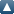# Items where Author is "Mukhigulashvili, Sulkhan"Up a level
 Export as ASCII CitationBibTeXDublin CoreEP3 XMLEndNoteHTML CitationJSONMETSObject IDsOpenURL ContextObjectRDF+N-TriplesRDF+N3RDF+XMLReferReference Manager
Group by: Item Type | No Grouping
Jump to: Article | Monograph
Number of items: 40.

## Article

Mukhigulashvili, Sulkhan and Manjikashvili, Mariam (2017) On one two-point BVP for the fourth order linear ordinary differential equation. Georgian Mathematical Journal, 24 (2). pp. 265-275.

Mukhigulashvili, Sulkhan (2016) The mixed BVP for second order nonlinear ordinary differential equation at resonance. Mathematische Nachrichten.

Mukhigulashvili, Sulkhan (2016) The mixed BVP for second order nonlinear ordinary differentialequation at resonance. Mathematische Nachrichten, 290 (2-3). pp. 393-400.

Mukhigulashvili, Sulkhan and Puza, Bedrich (2015) The Focal Boundary Value Problem For Strongly Singular Higher-Order Nonlinear Functional-Differential Equations. Boundary Value Problems.

Mukhigulashvili, Sulkhan (2015) The Nonlocal Boundary Value Problems For Strongly Singular Higher-Order Nonlinear Functional-Differential Equations. italian journal of pure and applied mathematics.

Mukhigulashvili, Sulkhan (2013) The Dirichlet Boundary Value Problems For Strongly Singular Higher-Order Nonlinear Functional-Differential Equations. Czechoslovak mathematical journal.

Mukhigulashvili, Sulkhan (2013) Nonlocal Boundary Value Problem For Strongly Singular Higher-Order Linear Functional-Differential Equations. electronic journal of qualitative theory of differential equations.

Mukhigulashvili, Sulkhan and Partsvania, Nino (2013) On one estimate for solutions of two-point boundary value problems for higher-order strongly singular linear differential equations. memoirs on differential equations and mathematical physics.

Mukhigulashvili, Sulkhan and Partsvania, Nino (2012) Two-point boundary value problems for strongly singular higher-order linear differential equations with deviating arguments. electronic journal of qualitative theory of differential equations.

Mukhigulashvili, Sulkhan (2011) The Dirichlet BVP The second Order Nonlinear Ordinary Differential Equation At Resonance. Italian Journal of Pure and Applied Mathematics.

Mukhigulashvili, Sulkhan and Puza, Bedrich and Partsvania, Nino (2011) On a periodic problem for higher-order differential equations with a deviating argument. Nonlinear Analysis.

Mukhigulashvili, Sulkhan (2010) On one problem with nonlinear boundary condition for systems of functional-differential equations. Differential equations.

Mukhigulashvili, Sulkhan and Puza, Bedrich and Kuzmych, Olena (2010) An optimal condition for the uniqueness of a periodic solution for systems of higher order linear functional differential equations. Miskolc Mathematical Notes.

Kiguradze, Ivan and Mukhigulashvili, Sulkhan (2009) On Periodic Solutions Of The System Of Two Linear Differential Equations. memoirs on differential equations and mathematical physics.

Mukhigulashvili, Sulkhan and Grytsay, Irina (2009) An Optimal Condition For The Uniqueness Of Periodic Solution For Linear Functional Differential Systems. electronic journal of qualitative theory of differential equations.

Mukhigulashvili, Sulkhan and Hakl, Robert (2009) A Periodic Boundary Value Problem For Functional Differential Equations Of Higher Order. georgian mathematical journal.

Lomtatidze, Alexander and Mukhigulashvili, Sulkhan and Sremr, Jiri (2008) Nonnegative Solutions of The Characteristic Initial Value Problem For Linear Partial Functional- Differential Equations of Hiperbolic Type. Mathematical and Computer Modelling.

Mukhigulashvili, Sulkhan and Puza, Bedrich (2007) On A Periodic Boundary Value Problem For \$3^{th}\$ Order linear Functional Differential Equations. Functional differential equations.

Mukhigulashvili, Sulkhan and Puza, Bedrich (2007) On A Periodic Boundary Value Problem For Cyclic Feetback Type Linear Functional Differential Systems. Memoirs on Differential Equations and Mathematical Physics.

Mukhigulashvili, Sulkhan and Hakl, Robert (2007) On A Periodic Boundary Value Problem For Third Order Linear Functional Differential Equations. memoirs on differential equations and mathematical physics.

Mukhigulashvili, Sulkhan (2007) On a Periodic Boundary Value Problem For Fourth Order Linear Functional Differential Equations. Georgian Mathematical Journal.

Mukhigulashvili, Sulkhan (2007) On a Priori Estimates of Solutions of Nonlinear Functional differential Inequalities of Higher Order With Boundary Conditions of Periodic Type. Memoirs on Differential Equations and Mathematical Physic.

Mukhigulashvili, Sulkhan (2007) On a periodic boundary value problem for third order linear functional differential equations. Nonlinear Analysis: Theory, Methods & Applications.

Mukhigulashvili, Sulkhan and Sremr, Jiri (2006) On A two-point Boundary Value Problem for Second Order Linear Functional Differential Equations With Monotone Operators. Functional Differential Equations.

Mukhigulashvili, Sulkhan (2006) On Periodic Solutions of Second Order Functional Differential Equations. Italian Journal of Pure and Applied Mathematics (20). pp. 29-50.

Mukhigulashvili, Sulkhan (2006) On a periodic boundary value problem for cyclic feedback type linear functional differential systems. Archiv der Mathematik.

Mukhigulashvili, Sulkhan (2006) On the solvability of the periodic problem for nonlinear second-order function-differential equations. Differential Equations.

Mukhigulashvili, Sulkhan and Hakl, Robert (2005) On A Boundary Value Problem For n -th Order Linear Functional Differential Systems. Georgian Mathematical Journal.

Mukhigulashvili, Sulkhan and Hakl, Robert (2005) On One Estimate For A Periodic Functions. Georgian Mathematical Journal .

Mukhigulashvili, Sulkhan (2005) On a periodic boundary value problem for second-order linear functional differential equations. Boundary Value Problems.

kiguradze, Ivan and Mukhigulashvili, Sulkhan (2005) On periodic solutions of two-dimensional nonautonomous differential systems. Nonlinear Analysis: Theory, Methods & Applications.

Mukhigulashvili, Sulkhan and Sremr, Jiri (2005) On the Solvability of the Dirichlet Problem for Nonlinear Second-Order Functional-Differential Equations. Differential Equations.

Kiguradze, Ivan and Mukhigulashvili, Sulkhan (2004) On Nonlinear Boundary Value Problems for Two-Dimensional Differential Systems. Differential Equations.

Mukhigulashvili, Sulkhan (2004) On the unique solvability of the Dirichlet problem for a second order linear functional differential equation. Differential Equations.

Mukhigulashvili, Sulkhan (2003) On two-point boundary value problems for two-dimensional differential systems with singularities. Georgian mathematicak journal.

Mukhigulashvili, Sulkhan and Lomtatidze, Alexander (1997) On a two-point boundary value problem for second order functional differential equations I. Memoirs on Differential Equations and Mathematical Physics.

Mukhigulashvili, Sulkhan and Lomtatidze, Alexandr (1997) On a two-point boundary value problem for second order functional differential equations II. Memoirs on Differential equations and Mathematical Physics.

Mukhigulashvili, Sulkhan (1995) On a two-point boundary value problem for second order functional differential equations. memoirs on differential equations and mathematical physicsmemoir.

Mukhigulashvili, Sulkhan and Lomtatidze, Alexander (1995) On periodic solutions of second order functional differential equations. Memoirs on differential equations and mathematical physics.

## Monograph

Mukhigulashvili, Sulkhan (2000) Two-point boundary value problems for second order functional differential equations. Other. Memoirs on Differential equations and Mathematical Physics.

This list was generated on Mon Jun 21 16:18:58 2021 PDT.